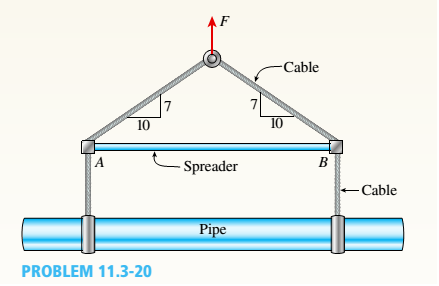Chapter 11, Problem 11.3.20P### Mechanics of Materials (MindTap Co...

9th Edition
Barry J. Goodno + 1 other
ISBN: 9781337093347
Textbook Problem

# The hoisting arrangement for lifting a large pipe is shown in the figure. The spreader is a steel tubular section with outer diameter 70 mm and inner diameter 57 mm. Its length is 2.6 m, and its modulus of elasticity is 200 GPa.Based upon a factor of safety of 2.25 with respect to Euler buckling of the spreader, what is the maximum weight of pipe that can be lifted? (Assume pinned conditions at the ends of the spreader.)To determine

The maximum weight of the pipe that can be lifted.

Explanation

Given information:

The outer diameter of the pipe is 70mm , the inner diameter of the pipe is 57mm , the length of the pipe is 2.6m , the modulus of the elasticity is 200GPa, and the factor of safety is 2.25 .

The figure below shows the free body diagram of hoisting arrangement.

Figure-(1)

Write the expression for the angle subtended by the spreader.

tanα=MNON ...... (I)

Here, the distance between point M to N is MN and the distance between point O to N is ON .

Write the expression for force acting along horizontal direction.

F hor=0pallow+Tcos(α)=0T=p allowcos(α) ...... (II)

Here, the horizontal force acting on spreader is Tcos(α) and the allowable load acting on the spreader is pallow .

Write the expression for force acting along vertical direction.

F ver=0Tsin(α)W max2=0 ...... (III)

Here, the vertical force acting on the spreader is Tsin(α) and maximum the weight of the pipe is Wmax .

Substitute pallowcos(α) for T in Equation (III).

p allowcos(α)sin(α)W max2=0p allowcos(α)sin(α)=W max2Wmax=2pallowtan(α) ...... (IV)

Write the expression for moment of inertia of circular pipe.

I=π64[( d 0)4( d i)4] ...... (V)

Here, the outer diameter of the pipe is d0 , the inside diameter of the pipe is di .

Write the expression for critical load acting on the spreader.

pcr=π2EIL2 ...... (VI)

Here, the young’s modulus of the pipe is E and the length of the pipe is L .

Write the expression for allowable load.

pallow=pcrn ...... (VII)

Here, the factor of safety is n .

Calculation:

Substitute 7 for MN and 10 for ON in Equation (I).

tan(α)=710tan(α)=0.7α=tan1(0.7)α=35°

Substitute 70mm for d0 and 57mm for di in Equation (V)

### Still sussing out bartleby?

Check out a sample textbook solution.

See a sample solution

#### The Answers to Your Study Problems

Solve them all with bartleby. Boost your grades with guidance from subject experts covering thousands of textbooks. All for just \$9.99/month

Get As ASAP

## Additional Engineering Solutions

#### What is an SPST switch?

Automotive Technology: A Systems Approach (MindTap Course List)

#### What is RADIUS? What advantage does it have over TACACS?

Principles of Information Security (MindTap Course List)

#### List and describe the different types of databases.

Database Systems: Design, Implementation, & Management

#### What is a DBMS, and what are its functions?

Database Systems: Design, Implementation, & Management

#### Explain the differences between a Gantt chart and a PERT/CPM chart

Systems Analysis and Design (Shelly Cashman Series) (MindTap Course List)